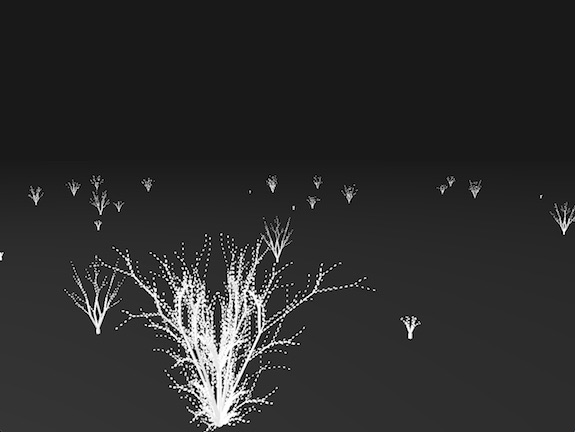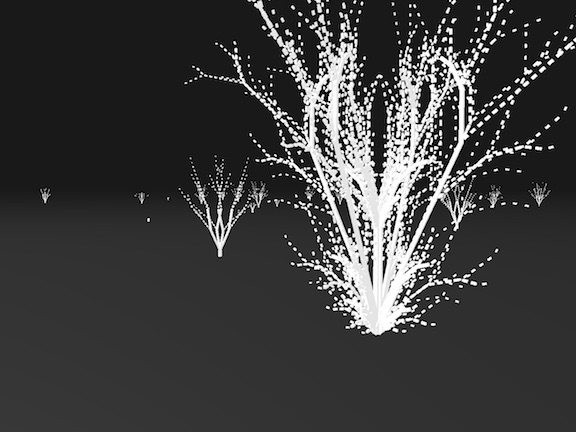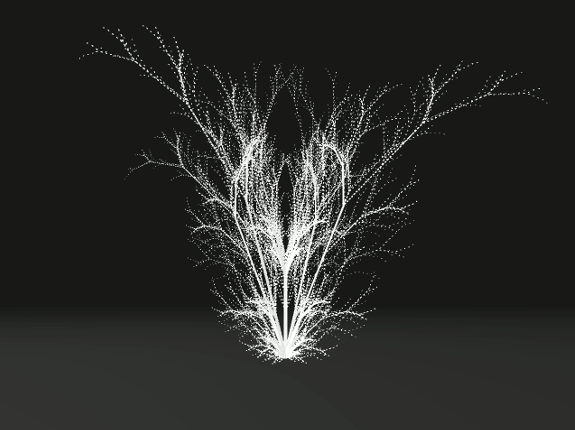## CPE471 Final Project by Daniel GriffithFor my final project in CPE 471, I really wanted to make procedurally generated objects. After learning about particle effects in Lab 11, I was fascinated with the idea of taking a single particle and having it leave a trail of particles to "draw" something. My original idea was to have the particle draw something like a flower, but I quickly realized that this was not something I could feasibly do in the amount of time allotted for the project. Instead I decided to use L-systems to create a scene filled with procedurally generated trees. For this project I used several techniques, including L-systems, blinn-phong shading, a free look camera, and some other techniques to create a scene filled with trees.

## Techniques Used

### L-Systems

To generate the trees in the scene I implemented several different L-system production rules, some of which I designed myself, others I found online. However, instead of having the upper portions of the tree drawn shorter than the lower portions of the tree, I inverted that convention and drew the lower parts of the tree much shorter than the upper parts of the tree, resulting in a "ghosting" effect where pieces of the tree are floating in air. To get different heights for the trees I varied the maximum generation of the L-system.

Since the L-systems were mostly locked in a single plane, to create 3D trees I ended up drawing each L-system 4 times, once for each of the 4 cardinal directions.

#### Custom Production Rules

Variables: Y F
Constants: + - [ ] # @
Start: Y
Rules: (Y -> F[#-Y@+Y]), (F -> FF)
Z Angle: 25°
X Angle: 5°
Here, F means "draw forward", - means "rotate positive 25° in the Z plane", + means "rotate negative 25° in the Z plane", # means "rotate positive 5° in the X plane", and @ means "rotate negative 5° in the X plane". Y does not correspond to a drawing action, but is used to control the evolution of the branches. The square bracket "[" corresponds with saving the current position and angles of the branch, which are restored with "]". The use of the square brackets allows for multiple branches from a single point. This is a custom set of rules I designed.Variables: X F
Constants: + - [ ]
Start: X
Rules: (X -> F-[[X]+X]+F[+FX]-X), (F -> FF)
Angle: 25°
Here, F means "draw forward", - means "rotate positive 25° in the Z plane", and + means "rotate negative 25° in the Z plane". X does not correspond to a drawing action, but is used to control the evolution of the branches. The square bracket "[" corresponds with saving the current position and angles of the branch, which are restored with "]". This set of rules can be found online at https://en.wikipedia.org/wiki/L-system.### Growth

To simulate the trees "growing", I limited the maximum generation drawn for each tree and set each tree on a timer which incremented every so many frames and increased the maximum generation the tree was drawn at. Once the tree was fully drawn I let the count increase 3 more times before resetting the maximum generation back to 0.

### Wind

To simulate a small wind in the forest of trees, I used a sinusoidal function to generate a small angular offset that I applied to the base component of each tree before drawing on each frame. Since the higher parts of the tree were drawn in relation to the base the rotation was applied to each piece.

## Sources

L-systems: https://en.wikipedia.org/wiki/L-system